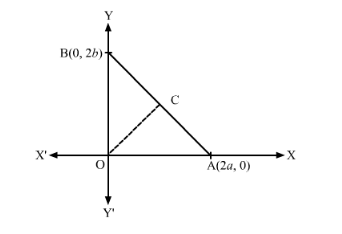# In Fig. 14.36, a right triangle BOA is given C is the mid-point of the hypotenuse AB.Question:

In Fig. 14.36, a right triangle BOA is given C is the mid-point of the hypotenuse AB. Show that it is equidistant from the vertices O, A  and B.Solution:

We have a right angled triangle $\Delta \mathrm{BOA}$, right angled at $\mathrm{O}$. Co-ordinates are $\mathrm{B}(0,2 b) ; \mathrm{A}(2 a, 0)$ and $\mathrm{C}(0,0)$.

We have to prove that mid-point C of hypotenuse AB is equidistant from the vertices.

In general to find the mid-point $\mathrm{P}(x, y)$ of two points $\mathrm{A}\left(x_{1}, y_{1}\right)$ and $\mathrm{B}\left(x_{2}, y_{2}\right)$ we use section formula as,

$\mathrm{P}(x, y)=\left(\frac{x_{1}+x_{2}}{2}, \frac{y_{1}+y_{2}}{2}\right)$

So co-ordinates of C is,

$\mathrm{C}(a, b)$

In general, the distance between $\mathrm{A}\left(x_{1}, y_{1}\right)$ and $\mathrm{B}\left(x_{2}, y_{2}\right)$ is given by,

$\mathrm{AB}=\sqrt{\left(x_{2}-x_{1}\right)^{2}+\left(y_{2}-y_{1}\right)^{2}}$

So,

$\mathrm{CO}=\sqrt{(a-0)^{2}+(b-0)^{2}}$

$=\sqrt{a^{2}+b^{2}}$

$\mathrm{CB}=\sqrt{(a-0)^{2}+(b-2 b)^{2}}$

$=\sqrt{a^{2}+b^{2}}$

$\mathrm{CA}=\sqrt{(a-2 a)^{2}+(b-0)^{2}}$

$=\sqrt{a^{2}+b^{2}}$

Hence, mid−point C of hypotenuse AB is equidistant from the vertices.